## Functional analysis front page

### Quick description

Functional analysis is the study of infinite-dimensional vector spaces. Typically these are spaces of functions such as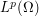being the space of functions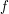where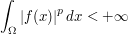for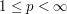. These spaces must have a topology, which is often given in terms of a norm such as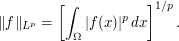The name comes from the notion of functionals which are continuous linear functions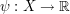where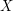is the space concerned. A major early problem was the identification of ways of representing the functionals of a given infinite dimensional space. For example, the Riesz representation theorem (or perhaps one should say theorems) says that if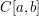is the space of continuous functions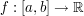, then any functional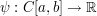, with the norm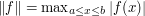on, could be represented in terms of a functionof bounded variation with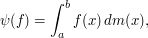where the integral is understood in the Riemann-Stieltjes sense. Related results concern, for example, the functionals onspaces.

Such analysis quickly led to the classification of infinite-dimensional spaces: Banach spaces, Hilbert spaces, reflexive spaces, and investigation of their properties.

This is a large area and involves large parts of classical and modern analysis. It is used in a large number of subjects, but especially partial differential equations, geometric analysis, quantum mechanics, and dynamical systems, to give a small sample of relatively applied parts of mathematics that use functional analysis.

### Prerequisites

Calculus, basic analysis.

### General discussion

Here is a (small) sample of topics:

• representation of functionals

• dual spaces, topologies

• different notions of convergence: strong, weak, weak*

• topological vector spaces

• operator algebras

• spaces of (linear) operators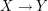• geometry of Banach spaces

• spaces of measures with values in (Banach) spaces (see vector measure)

• existence of solutions to operator equations: solve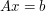for• fixed point and minimax theorems

• Fredholm operators and Fredholm index

• compactness of sets in specific spaces

• optimization of functions defined on spaces (see Convex and variational analysis)

• differential equations on spaces and evolution equations

• convex and variational analysis

• interpolation spaces

• particular (classes of) spaces: e.g., Sobolev spaces, Orlicz spaces,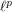,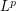,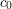### Expand section...

Hi,

First of all, an excellent site! It's great to have such a compendium of mathematical knowledge online, accessable to all. So a great deal of thanks goes to the creators of this site!

I'm in Year 12 in Australia and I just stumbled upon this page [on functional analysis], and I'd love to see it expanded. It looks to be an interesting field! I assume that capital Omega here is a space/topology, maybe even a set being integrated over? Could an explanation be given of the theory behind L^p(Omega) spaces and how it ties in more broadly to this topic?

I look forward to hearing further (and just as a side-note, I believe my mathematical background to be good so far - I have a good understanding of convergence of sequences, Maclaurin series, complex numbers, derivatives of inverse trigonometric functions, etc). I have read a little of analysis, to which I have been impressed so far, but that I have only broadly skimmed.

Thanks once again.
Davin

## Post new comment

(Note: commenting is not possible on this snapshot.)

Before posting from this form, please consider whether it would be more appropriate to make an inline comment using the Turn commenting on link near the bottom of the window. (Simply click the link, move the cursor over the article, and click on the piece of text on which you want to comment.)